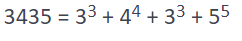×

Search anything:

# Munchausen Number

#### Algorithms List of Mathematical AlgorithmsGet this book -> Problems on Array: For Interviews and Competitive Programming

A number that is equal to the sum of its digits each raised to the power equal to its digit is a Munchausen Number or perfect digit-to-digit invariant(PDDI).

For example :1.In above example each digit raised to itself on addition equals to number itself.

2.Another number which exhibits this property is 1 .

## Pseudocode

So a simple algorithm for identification of Munchausen Number is as follows:

• For a given input number break it into constituent digits.
• Find the sum of each of its digits raised to itself.
• If it is equal to the original number,then it is the required Munchausen number.

## Implementation

For example let's try to find if a number is a Munchausen number or not?
We make use of our psudeo code for breaking the problem.

The Cpp implementation is as follows :

``````#include<bits/stdc++.h>
using namespace std;
int pwr; //global variable
bool isMunchausen(int n) {
//Precomputing i raised to power i for every i (0-9)
for (int i = 0; i <= 9; i++ )
{
pwr[i] = pow( i,i );
}
int sum = 0;
int temp = n;
while (temp)
{
sum =sum + pwr[(temp % 10)];
temp =temp/10;
}
return (sum == n);
}
int main() {
int n=1000;

if(isMunchausen(n))//function call
{cout<<"YES"<<endl;}
else
{cout<<"NO"<<endl;}
return 0;
}
``````

## Output

``````NO
``````

Ex 2 : Now let's try to find over a range of numbers
We extend the same approach used over a set of numbers to get the desired result .
The Cpp implementation of it is as follows:

``````#include <bits/stdc++.h>
using namespace std;
int pwr; //global variable for accessing the values
void printNumbers(int n)
{
//Precomputing i raised to power i for every i (0-9)
for (int i = 0; i <= 9; i++ )
{
pwr[i] = pow( i,i );}
// checking up to n
for (int i = 1; i <= n; i++)
{
if (isMunchausen(i))
cout << i << "\n"; //if true prints the number
}
}
bool isMunchausen(int n) {
int sum = 0;
int temp = n;

while (temp) {
sum =sum + pwr[(temp % 10)]; //Adding the units digit to the count
temp =temp/10; //Breaking the number for next higher power extraction
}
return (sum == n);
}

int main() {
int n = 10000; //change here for computing other numbers
printNumbers(n);
return 0;
}
``````

## Output

``````1
3435
``````

In above examples 0^0=0 is considered.

# Conclusions :

There are a very few numbers greater than zero which are Munchausen numbers or PDDI like 1 , 3435 and 438579088. The gap widens so on so forth from our observation.